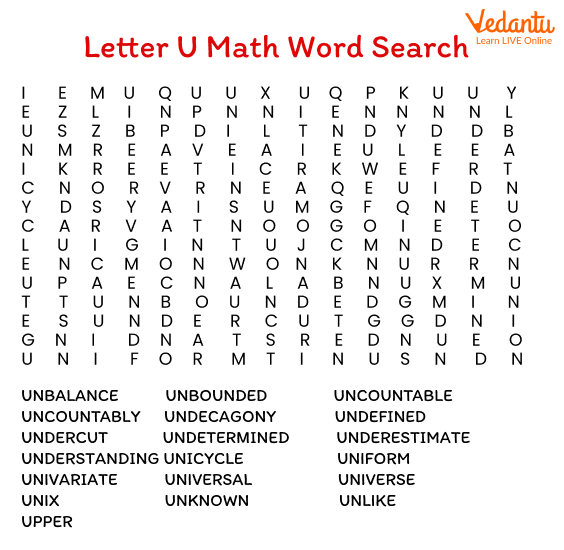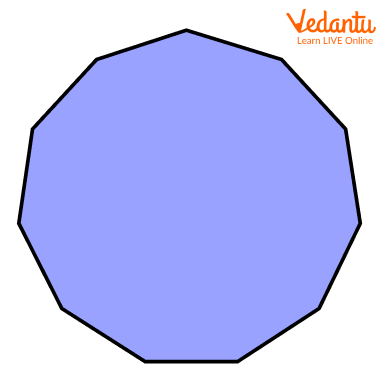Courses
Courses for Kids
Free study material
Free LIVE classes
MoreLIVE
Join Vedantu’s FREE Mastercalss

Maths is one subject with lots of recurring terms and abbreviations, which every child cannot get in one go. Also, without knowing these terms, it's really difficult to understand certain Maths concepts and solve problems. So, what's the solution?

We are here to make this challenging task of learning new Maths words easier for children by creating a Maths glossary. In this article, we will learn Maths words that start with U. We have also included a brief description of each of them to help you remember them easily!

## 10 Maths Words Starting with the Letter UMaths vocabulary – words starting with ‘u’

1. Unit: It is a standard quantity used in the measurement. For example, the universal unit of length in metric is the meter, while the units of time are second, minute, hour, etc.

2. Unitary Method: This is a Mathematical method used in algebra to solve problems involving quantity, where the given quantity is first calculated with respect to ‘one’ unit.

3. Unequal: In Mathematics, unequal represents the terms, sets, or conditions which are not equal. It is denoted by '≠'.

4. Undefined Term: In Maths, if the denominator of a fraction is 0, then that term/fraction is called undefined and cannot be solved.

5. Unlike Fractions: These are fractions with different denominators, no matter what is the numerator. For example, the fractions 11/5 and 5/19 are unlike fractions.

6. Unit Fraction: A fraction that has 1 in its numerator and any natural number in its denominator. Note that the denominator cannot be 0 or negative integer. For example, 1/8, 1/5, etc.

7. Undecagon: It is a 2-D polygon with 11 sides and 11 angles. It is also called Hendecagon.An undecagon

1. Unknown Variable: In algebra, a variable (usually x, y, or z) is called unknown when its value is not known. We need to find its value.

2. Unlike Terms: In algebra, unlike terms are defined as the ones which contain different variables or the same variables with different exponents. For example, 3x + 3x2, 4x3 + 5y4, etc.

3. Universal Set: A set that contains all the elements of the given sets, without any repetition of elements is called a universal set. It is denoted using the symbol U. For example, if A and B are two sets such that A = {1, 2, 3, c} and B = {a, b, c}, then the universal set is given by U = {1, 2, 3, a, b, c}.

## Conclusion

These Maths dictionary words starting with the letter ‘u’ introduce kids to many important words and make it really easy for them to memorise. However, it can sometimes become confusing to learn so many words together. So, students should keep repeating words until they become clear.

Last updated date: 21st Sep 2023
Total views: 138k
Views today: 2.38k

1. How can a Mathematics glossary help students?

As students progress to higher classes, they are introduced to many advanced concepts with several new words and phrases. So, learning such common Maths words starting with different letters will help students to understand the concepts clearly. It will also aid students in solving Mathematical problems easily.

2. What is the meaning of ‘U’ in different branches of Mathematics?

• Set theory: The symbol U represents the union of two sets.

• Geometry: A unit circle is a circle on the x—y plane with radius 1 and centre as the origin.

• Algebra: A unit vector in Maths is a vector of magnitude 1. Generally, û is a unit vector with the same direction as vector u.

• Probability: The symbol U represents the uniform distribution; for example, U(a,b), which means equal probability in range a, b.